### 【Python】淺談梯度下降與實作(中)：初階的變形者們

========================================

TeX All the Things
2. 本文中有些許高中數學，不會太硬，請放心咀嚼。

========================================

$$w_k^{(j+1)}=w_k^{(j)}-\alpha\nabla Loss(w_k) ... (1)$$

$$j 為迭代次數，\alpha 為學習率，k為第k個權重$$

$$W^{(j+1)}=W^{(j)}-\alpha\times\frac{2}{n}\sum_{i=1}^{n}{X^i\left(W^{(j)}X^i-Y_t^i\right)} ... (2)$$

$$i 為資料編號，j 為迭代次數，n為資料筆數，\alpha為學習率$$

SGD 的概念是：「每次隨機取一筆資料來算，反復計算以得到最佳結果。」

$$W^{(j+1)}=W^{(j)}-\alpha\times 2X^i\left(W^{(j)}X^i-Y_t^i\right) ... (3)$$

$$i 為資料編號，j 為迭代次數，\alpha 為學習率$$

# -*- coding: utf-8 -*-
# 引用部分上一章之程式碼
#
# Shayne, 2019.10.09

import numpy as np
import matplotlib.pyplot as plt

# 給定隨機種子，使每次執行結果保持一致
np.random.seed(1)

def getdata(n):
# n為產生資料量
x = np.arange(-5, 5.1, 10/(n-1))
# 給定一個固定的參數，再加上隨機變動值作為雜訊，其變動值介於 +-10 之間
y = 3*x + 2 + (np.random.rand(len(x))-0.5)*20
return x, y

def plot_error(x, y):
a = np.arange(-10, 16, 1)
b = np.arange(-10, 16, 1)
mesh = np.meshgrid(a, b)

sqr_err = 0
for xs, ys in zip(x, y):
sqr_err += ((mesh*xs + mesh) - ys) ** 2
loss = sqr_err/len(x)

plt.contour(mesh, mesh, loss, 20, cmap=plt.cm.jet)
plt.xlabel('a')
plt.ylabel('b')
plt.axis('scaled')
plt.title('function loss')

# 實作 BGD
class my_BGD:
def __init__(self, a, b, x, y, alpha):
self.a = a
self.b = b
self.x = x
self.y = y
self.alpha = alpha

self.a_old = a
self.b_old = b

self.loss = None;

# Loss function
def mse(self):
sqr_err = ((self.a*self.x + self.b) - self.y) ** 2
return np.mean(sqr_err)

grad_a = 2 * np.mean((self.a*self.x + self.b - self.y) * (self.x))
grad_b = 2 * np.mean((self.a*self.x + self.b - self.y) * (1))

def update(self):
# 計算梯度

# 梯度更新
self.a_old = self.a
self.b_old = self.b
self.a = self.a - self.alpha * grad_a
self.b = self.b - self.alpha * grad_b
self.loss = self.mse();

1. 把原本 BGD 的 np.mean 拿掉。

2. 新增隨機抽取資料的過程。

# 實作 SGD
class my_SGD(my_BGD):
def __init__(self, a, b, x, y, alpha):
super().__init__(a, b, x, y, alpha)

# 隨機取一筆資料進行計算
idx = np.random.randint(len(x))

grad_a = 2 * (self.a*self.x[idx] + self.b - self.y[idx]) * (self.x[idx])
grad_b = 2 * (self.a*self.x[idx] + self.b - self.y[idx]) * (1)
return grad_a, grad_b

# 隨機產生一組資料
x, y = getdata(51)

# 繪製誤差空間底圖
plot_error(x, y)

# 初始設定
alpha = 0.05

# 從 -9 開始，能看得更明顯
a = -9; b = -9

# 類別 SGD 初始化
mlclass = my_SGD(a, b, x, y, alpha) # <=== 後續的主程式，只要改這句話就好！

plt.plot(a, b, 'ro-')
plt.title('Initial, loss='+'{:.2f}'.format(mlclass.mse())+'\na='+
'{:.2f}'.format(a)+', b='+'{:.2f}'.format(b))

# 開始迭代
for i in range(1, 11):
mlclass.update()
print('iter='+str(i)+', loss='+'{:.2f}'.format(mlclass.loss))

plt.plot((mlclass.a_old, mlclass.a), (mlclass.b_old, mlclass.b), 'ro-')
plt.title('iter='+str(i)+', loss='+'{:.2f}'.format(mlclass.loss)+'\na='+
'{:.2f}'.format(mlclass.a)+', b='+'{:.2f}'.format(mlclass.b))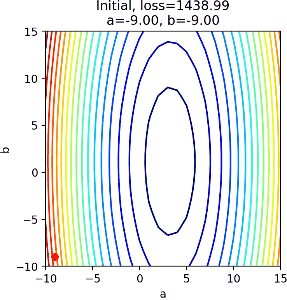請讀者自行將數據量增大，就能觀察到迭代速度的差異。

$$W^{(j+1)}=W^{(j)}-\alpha\times 2X^i\left(W^{(j)}X^i-Y_t^i\right) ... (3)$$

$$i 為資料編號，j 為迭代次數，\alpha為學習率$$

$$W^{(j+1)}=W^{(j)}-\alpha\times\frac{2}{m}\sum_{i=1}^{m}{X^i\left(W^{(j)}X^i-Y_t^i\right)} ... (4)$$

$$i 為資料編號，j 為迭代次數，m為資料筆數，\alpha為學習率$$

MBGD 目前在深度學習中是主流的算法，後續的其他演化多是基於 MBGD 的小批量概念。

1. 代入 batch_size 參數，限制每次迭代的資料數量，並修改計算損失與梯度的函數。

2. 需要新增更新批次資料的方法(update_batch)，每次迭代後，都要換上一批新的資料。

# 請注意：
# 本類別不針對資料跑完後做"任何處理"，即跑到最後若超出陣列範圍，loss 會變成 nan。
#
# Lazy Shayne, 2019.10.09

class my_MBGD(my_BGD):
def __init__(self, a, b, x, y, alpha, batch_size):
super().__init__(a, b, x, y, alpha)

self.idx = 0
self.batch_size = batch_size

# 使用 np.random.permutation 給定資料取出順序
self.suffle_idx = np.random.permutation(len(x))
self.update_batch();

# 更新批次
def update_batch(self):
#每次更新時，採滾動的方式依次取出 N 筆資料
idx = self.suffle_idx[self.idx:self.idx+self.batch_size];
self.idx += self.batch_size

self.x_batch = self.x[idx]
self.y_batch = self.y[idx]

# Loss function
def mse(self):
sqr_err = ((self.a*self.x_batch + self.b) - self.y_batch) ** 2
return np.mean(sqr_err)

grad_a = 2 * np.mean((self.a*self.x_batch + self.b - self.y_batch) * (self.x_batch))
grad_b = 2 * np.mean((self.a*self.x_batch + self.b - self.y_batch) * (1))
self.update_batch();
return grad_a, grad_b

# 參數設定
alpha = 0.05
batch_size = 5

# 類別 MBGD 初始化
mlclass = my_MBGD(a, b, x, y, alpha, batch_size)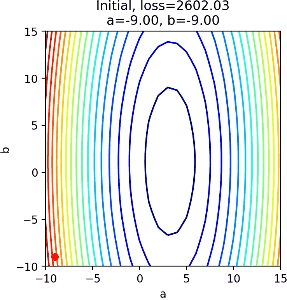## 三、動量(momentum)

$$W^{(j+1)}=W^{(j)}-\alpha\nabla L(W^{(j)})+\gamma(W^{(j)}-W^{(j-1)})... (5)$$

$$V^{(j)}=\gamma V^{(j-1)}+\alpha\nabla L(W^{(j)})$$

$$W^{(j+1)}=W^{(j)}-V^{(j)}... (5.2)$$

$$其中，\nabla L(W^{(j)})=\frac{2}{m}\sum_{i=1}^{m}{X^i\left(W^{(j)}X^i-Y_t^i\right)}$$

$$i 為資料編號，j 為迭代次數，m為資料筆數，\alpha為學習率，\gamma為動量參數$$

對照 (5) 式與 (5.2) 式，$V^0=\alpha G^0$

代換上式的$(W^1-W^0)$，可得$W^2=W^1-\alpha G^1+\gamma(-\alpha G^0)$

再對照一次，$V^1=\alpha G^1+\gamma V^0$
代換$V^0$後，得$V^1=\alpha G^1+\gamma\alpha G^0$

代換上式的$(W^2-W^1)$，可得$W^3=W^2-\alpha G^2+\gamma(-\alpha G^1+\gamma(-\alpha G^0))$

最後一次對照，$V^2=\alpha G^2+\gamma V^1$
代換$V^1$後，得$V^2=\alpha G^2+\gamma\alpha G^1+(\gamma)^2\alpha G^0$參考資料：深度学习优化函数详解（4）-- momentum 动量法

SGD 和 SGDM 在$W^0$走到$W^1$的過程是一樣的，之後則有動量的差異而產生分歧。

class my_SGDM(my_MBGD):
def __init__(self, a, b, x, y, alpha, batch_size, gamma):
super().__init__(a, b, x, y, alpha, batch_size)

# Momentum parameter
self.gamma = gamma

# 動量累加項
self.sum_Ma = 0
self.sum_Mb = 0

def update(self):
self.a_old = self.a
self.b_old = self.b

# 計算梯度

# 動量累加
self.sum_Ma = self.gamma * self.sum_Ma + self.alpha * grad_a
self.sum_Mb = self.gamma * self.sum_Mb + self.alpha * grad_b

# 梯度更新
self.a -= self.sum_Ma
self.b -= self.sum_Mb

self.loss = self.mse();


# 參數設定
alpha = 0.01
batch_size = 5
gamma = 0.8

# 類別 SGDM 初始化
mlclass = my_SGDM(a, b, x, y, alpha, batch_size, gamma)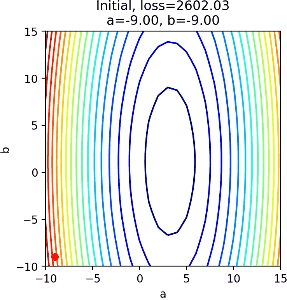## 四、NAG

NAG 是 Nesterov accelerated gradient 的縮寫。

$$V^{(j)}=\gamma V^{(j-1)}+\alpha\nabla L(W^{(j)}-\gamma V^{(j-1)})$$

$$W^{(j+1)}=W^{(j)}-V^{(j)}... (6)$$

NAG 大部分的東西都和 SGDM 相同，我看這次就不要再推公式了，因此直接畫張圖吧！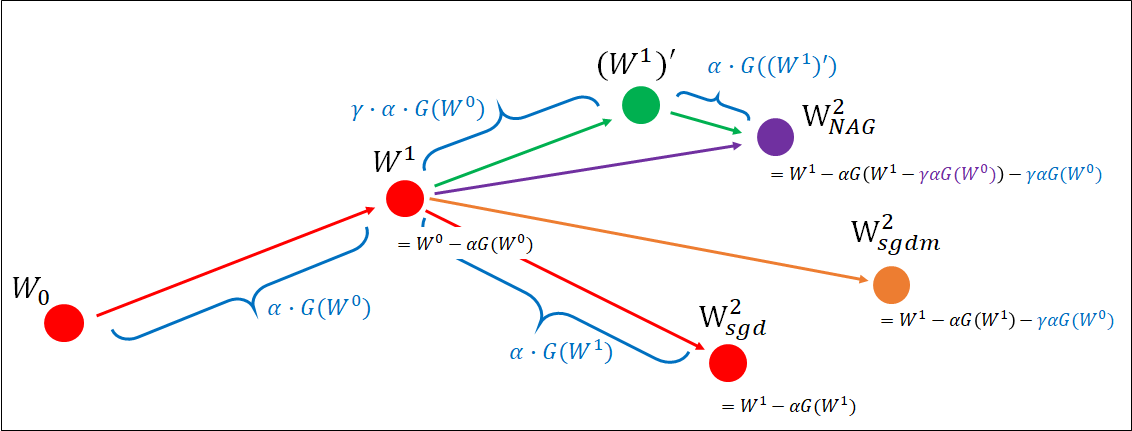參考資料：深度學習優化函數詳解（5）-- Nesterov accelerated gradient (NAG)

NAG 和 SGDM 在$W^0$走到$W^1$的過程也是一樣的。

「多往前走一些」就是 NAG 最大的特色。

class my_NAG(my_SGDM):
def __init__(self, a, b, x, y, alpha, batch_size, gamma):
super().__init__(a, b, x, y, alpha, batch_size, gamma)

# 往前多走一點
a = self.a - self.sum_Ma
b = self.b - self.sum_Mb

grad_a = 2 * np.mean((a*self.x_batch + b - self.y_batch) * (self.x_batch))
grad_b = 2 * np.mean((a*self.x_batch + b - self.y_batch) * (1))
self.update_batch();
return grad_a, grad_b

# 參數設定
alpha = 0.01
batch_size = 5
gamma = 0.8

# 類別 NAG 初始化
mlclass = my_NAG(a, b, x, y, alpha, batch_size, gamma)

##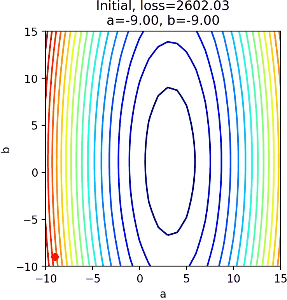【Python】淺談梯度下降與實作(下)：猙獰的變形者們